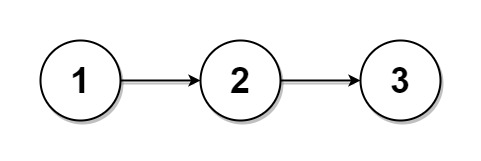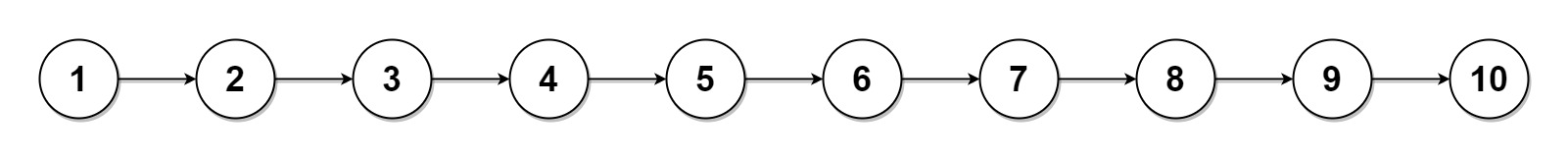## Algorithm

Problem Name: 725. Split Linked List in Parts

Given the `head` of a singly linked list and an integer `k`, split the linked list into `k` consecutive linked list parts.

The length of each part should be as equal as possible: no two parts should have a size differing by more than one. This may lead to some parts being null.

The parts should be in the order of occurrence in the input list, and parts occurring earlier should always have a size greater than or equal to parts occurring later.

Return an array of the `k` parts.

Example 1:```Input: head = [1,2,3], k = 5
Output: [,,,[],[]]
Explanation:
The first element output has output.val = 1, output.next = null.
The last element output is null, but its string representation as a ListNode is [].
```

Example 2:```Input: head = [1,2,3,4,5,6,7,8,9,10], k = 3
Output: [[1,2,3,4],[5,6,7],[8,9,10]]
Explanation:
The input has been split into consecutive parts with size difference at most 1, and earlier parts are a larger size than the later parts.
```

Constraints:

• The number of nodes in the list is in the range `[0, 1000]`.
• `0 <= Node.val <= 1000`
• `1 <= k <= 50`

## Code Examples

### #1 Code Example with C++ Programming

```Code - C++ Programming```

``````
class Solution {
public:
vector splitListToParts(ListNode* root, int k) {
vectorres;
int len = 0;
ListNode* head = new ListNode(0), *pre = root, *cur = head, *next, *p = root;
while(p && ++len) p = p->next;
int m = len % k, l = len / k;
while(k--){
cur->next = pre;
for(int i = 0; i < l; i++) cur = cur->next;
if(m) cur = cur->next, m--;
next = cur->next;
cur->next = NULL;
res.push_back(pre);
pre = next;
}
return res;
}
};
``````
Copy The Code &

Input

cmd
head = [1,2,3], k = 5

Output

cmd
[,,,[],[]]

### #2 Code Example with Java Programming

```Code - Java Programming```

``````
class Solution {
public ListNode[] splitListToParts(ListNode head, int k) {
ListNode[] arr = new ListNode[k];
int nodeLength = 0;
for (ListNode curr = head; curr != null; curr = curr.next) {
nodeLength++;
}
int n = nodeLength / k;
int remaining = nodeLength % k;
ListNode prev = null;
for (int i = 0; i < k && head != null; i++, remaining--) {
for (int j = 0; j < n + (remaining > 0 ? 1 : 0); j++) {
}
prev.next = null;
}
return arr;
}
}
``````
Copy The Code &

Input

cmd
head = [1,2,3], k = 5

Output

cmd
[,,,[],[]]

### #3 Code Example with Javascript Programming

```Code - Javascript Programming```

``````
const splitListToParts = function(root, k) {
let cur = root;
let N = 0;
while (cur != null) {
cur = cur.next;
N++;
}
let width = Math.floor(N / k),
rem = N % k;
let ans = [];
cur = root;
for (let i = 0; i < k; ++i) {
for (let j = 0; j < width + (i < rem ? 1 : 0) - 1; ++j) {
if (cur != null) cur = cur.next;
}
if (cur != null) {
let prev = cur;
cur = cur.next;
prev.next = null;
}
}
return ans;
};
``````
Copy The Code &

Input

cmd
head = [1,2,3,4,5,6,7,8,9,10], k = 3

Output

cmd
[[1,2,3,4],[5,6,7],[8,9,10]]

### #4 Code Example with Python Programming

```Code - Python Programming```

``````
class Solution:
def splitListToParts(self, root: ListNode, k: int) -> List[ListNode]:
n = 0
node = root
while node:
n += 1
node = node.next
count = n // k
residual = n % k

i = 0
ret = [[] for _ in range(k)]
prev = root
while prev and k > 0:
node = prev
leftover = count
ret[i] = node
i += 1
while node and leftover > 1:
node = node.next
leftover -= 1
if node and count != 0 and residual:
node = node.next
residual -= 1
prev = node.next if node else None
if node:
node.next = None
k -= 1
return ret
``````
Copy The Code &

Input

cmd
head = [1,2,3,4,5,6,7,8,9,10], k = 3

Output

cmd
[[1,2,3,4],[5,6,7],[8,9,10]]